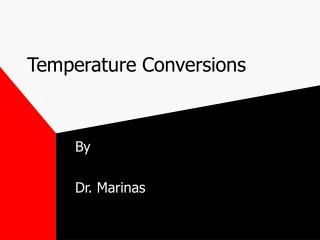DownloadDownload PresentationTemperature Conversions

# Temperature Conversions

Télécharger la présentation## Temperature Conversions

- - - - - - - - - - - - - - - - - - - - - - - - - - - E N D - - - - - - - - - - - - - - - - - - - - - - - - - - -
##### Presentation Transcript

1. Temperature Conversions By Dr. Marinas

2. Fahrenheit and Celsius

3. Fahrenheit/Celsius Formulas • C= 5/9 (F - 32) • Find the Celsius temperature for 212 degrees Fahrenheit • C=5/9(212 - 32) • C = 5/9 (180) • C = 100 degrees

4. Celsius, Kelvin, Fahrenheit

5. Celsius/Kelvin Formulas • K = C + 273.15 • What is the Kelvin temperature that corresponds to 100 degrees Celsius? • K = 100 + 273.15 • K = 373.15 degrees

6. Fahrenheit to Celsius C= 5/9 (F - 32) Celsius to Kelvin K= C + 273.15 Substitute the value of C from the first equation into the second equation K = [5/9 (F - 32)] + 273.15 Fahrenheit to Kelvin Formula